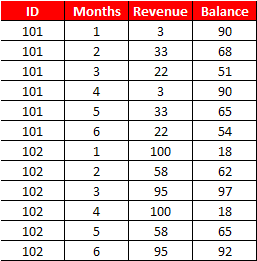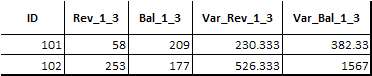# Intermediate Proc SQL Tutorial

Example 1 : Count Cases Where Condition is TRUE

The input data is shown below. Suppose you are asked to calculate the number of Ys and Ns of column Z by column X.Intermediate SQL Tutorial

data xyz;
input x y\$ z\$;
cards;
1 23 Y
1 24 N
1 25 Y
2 21 Y
2 22 Y
3 25 N
3 36 Y
;
run;
proc sql noprint;
create table tt as
select x,
sum(case when z= "Y" then 1 else 0 end) as z_Y,
sum(case when z= "N" then 1 else 0 end) as z_N
from xyz
group by x;
quit;

Example 2 : Creating trend variablesSample Dataset
data example1;
input ID Months Revenue Balance;
cards;
101 1 3 90
101 2 33 68
101 3 22 51
101 4 3 90
101 5 33 65
101 6 22 54
102 1 100 18
102 2 58 62
102 3 95 97
102 4 100 18
102 5 58 65
102 6 95 92
;
Task : Calculate total revenue and total balance accumulated in the first 3 months and how much the data spreads in the first 3 months time period.
proc sql noprint;
create table output1 as
select ID,
sum(case when 1 <= Months <= 3 then Revenue else . end) as Rev_1_3,
sum(case when 1 <= Months <= 3 then Balance else . end) as Bal_1_3,
var(case when 1 <= Months <= 3 then Revenue else . end) as Var_Rev_1_3,
var(case when 1 <= Months <= 3 then Balance else . end) as Var_Bal_1_3
from example1
group by ID;
quit;

Output :Output
Detailed Code for Multiple Variables

Example 3 : Check the Status Flag between the start date and end date

DATA dates;
INPUT ID Period : date9. Status \$;
FORMAT Period date9.;
CARDS;
1 13may2000 Y
1 17oct1999 Y
1 03feb2001 N
1 28feb2001 N
2 10nov2000 Y
2 25apr2001 N
3 03jun1997 Y
3 14jan2001 Y
3 24oct1998 N
3 27aug2000 N
;
RUN;
proc sql;
select a.ID , a.Period as Start_Date, b.Period as End_Date,
a.Status as Status_Start, b.Status as Status_End
from dates a left join
(select * from dates group by ID having Period = max(Period)) b
on a.ID = b.ID
group by a.ID
having a.Period = min(a.Period);
quit;

Example 4 : Calculate Percentage Change between the first and last row
data temp;
input ID time \$ x1-x3;
cards;
1 Y1 85 85 86
1 Y2 80 79 70
1 Y3 78 77 87
2 Y1 79 79 79
2 Y2 83 83 85
;
run;
data temp2;
set temp;
retain Serial 0;
If first.ID then Serial = 1;
else Serial = Serial + 1;
by ID;
run;
proc sql noprint;
create table t2 as
select a.ID, (a.x1-b.x1)/b.x1 *100 as change
from temp2 a left join
(select ID, x1 from temp2
group by ID
having serial = min(serial)) b
on a.ID = b.ID
group by a.ID
having serial = max(serial);
quit;

Example 5 : Extract First and Last Observation within a Group

PROC SQL : Alternative to First. Statement
proc sql;
create table output2 (drop=n) as
select *, monotonic() as n
from example1
group by ID
having min(n) = n;
quit;
PROC SQL : Alternative to Last. Statement
proc sql;
create table output2 (drop=n) as
select *, monotonic() as n
from example1
group by ID
having max(n) = n;
quit;
Example 6 : Self Join
Suppose you have data for employees. It comprises of employees' name, ID and manager ID. You need to find out manager name.Proc SQL : Self Join
data example2;
input Name \$ ID ManagerID;
cards;
Smith 123 456
Robert 456 .
William 222 456
Daniel 777 222
Cook 383 222
;
run;
SQL Query : Self Join
proc sql;
create table want as
select a.*, b.Name as Manager
from example2 as a left join example2 as b
on a.managerid = b.id;
quit;
Example 7 : Capping Extreme Values
Suppose you need to cap values of a column.

data have1;
input x y z;
cards;
101  1 10
102  2 20
103  3 45
104  1 23
105  2 42
106  3 46
107  1 61
109  2 22
110  3 28
111  1 30
112  2 32
113  3 39
;
run;
proc sql noprint;
create table output2 (drop=z rename= (z1=z))  as
select *, case when z >=  40 then 40 else z
end as z1 from have1;
quit;
First cap values of column z and then sum all the z values by column y.
proc sql;
select sum(z1) as OutCol
from (select *, case when z >=  40 then 40 else z
end as z1 from have1)
group by y;
quit;

Example 8 : Select All Excluding 1 Variable
proc sql;
create table want (drop=x) as
select a.*,b.*
from dat1 a, dat2 (rename=(id=x)) b
where a.id = b.x;
quit;
Example 9 : Sub Query - ANY and ALL Operators

1. ANY operator selects values that pass the comparison test with any of the values that are returned by the sub-query.

2. ALL operator selects values that pass the comparison test with all of the values that are returned by the sub-query.
data jansale;
input sale id;
cards;
100 1
105 2
108 3
110 4
;
run;
data febsale;
input sale id;
cards;
120 1
105 2
118 3
117 4
;
run;
proc sql;
select *
from jansale
where sale < all (select sale from febsale);
quit;
proc sql;
select *
from jansale
where sale < any (select sale from febsale);
quit;Related PostsDeepanshu founded ListenData with a simple objective - Make analytics easy to understand and follow. He has over 8 years of experience in data science. During his tenure, he has worked with global clients in various domains like Banking, Insurance, Telecom and Human Resource.

3 Responses to "Intermediate Proc SQL Tutorial"
1.This comment has been removed by the author.

2.This comment has been removed by the author.

3.hello , in code 2 , what does revenue else . end means ? Also i am unable to download detaled code file , as it is password protected .can you please share the password.

Next → ← Prev
Love this Post? Spread the Word!
Share